# What data scientist should know?

In this blog post, I will try to give you the first 10 things to become a Data Scientist .

For sure, depending of your background, you should learn many others things needed to become a great Data Scientist.

This is my personal list of the things that as data scientist should know:

I should remark that I am missing many other import programming languages that can be used in Data Science such as R, Spark, Scala, Ruby , JavaScript, Go and Swift and tools of ingestion of Data such as Apache Kafka and creation of the Cloud Infrastructure such as Terraform with Terragrunt and for the automatization of the ETL , Airflow and Jenkins and for sure the CLI in Linux and the use of Git and Unit test to check your programs. In addition I am skipping all the part of Deep Learning in details and Machine Learning in the Cloud that will be subject of future posts.

I know there are a toons of things that is needed to know to become a great Data Scientist but I will introduce only the essential topics based on Python and a little of SQL to manage the data from Cloud Databases.

You have also to know basis of Data Engineering such as in the previous post here and a little of Mathematics to understand how to solve the problems first by creating your algorithms which solves what you want to produce and analyze.

I have collected the information from different sources among them: Google , Udemy , Coursera, DataCamp , Pluralsight and EdX.

# Python for Data SciencePython Operator Precedence

From Python documentation on operator precedence (Section 5.15)

Highest precedence at top, lowest at bottom. Operators in the same box evaluate left to right.

Operator Description
() Parentheses (grouping)
f(args…) Function call
x[index:index] Slicing
x[index] Subscription
x.attribute Attribute reference
** Exponentiation
~x Bitwise not
+x, -x Positive, negative
*, /, % Multiplication, division, remainder
«, » Bitwise shifts
& Bitwise AND
^ Bitwise XOR
| Bitwise OR
in, not in, is, is not, <, <=, >, >=, <>, !=, == Comparisons, membership, identity
not x Boolean NOT
and Boolean AND
or Boolean OR
lambda Lambda expression

## Types and Type Conversion

str()
'5', '3.45', 'True' #Variables to strings

int()
5, 3, 1 #Variables to integers

float()
5.0, 1.0 #Variables to floats

bool()
True True True , , #Variables to boolean


### Libraries

Data analysis -> pandas

Scientific computing -> numpy

2D plotting -> matplotlib

Machine learning -> Scikit-Learn

### Import Libraries

import numpy
import numpy as np


### Selective import

from math import pi


### Strings

 my_string = 'thisStringisAwesome'
my_string
'thisStringisAwesome'



String Operation

 my_string * 2
'thisStringisAwesomethisStringisAwesome'
my_string +'Innit'
'thisStringisAwesomeinnit'
'm' in  my_string
True


String Indexing

Index starts at 0

 my_string
my_string[4:9]


String Methods

 my_string.upper() #String to uppercase
my_string.lower() #String to lowercase
my_string.count('w') #Count String elements
my_string.replace('e','i') #Replace String elements
my_string.strip() #Strip whitespoces


### Lists

 my_list = [1, 2, 3, 4]
my_array = np.array(my_list)
my_2darray = np.array([[1,2,3],[4,5,6]])



Selecting Numpy Array Elements

Index starts at 0

Subset
my_array #Select item at index 1
2
Slice
my_array[ 0:2]#Select items at index 0 and 1
array([1, 2])
Subset 2D Numpy arrays
my_2darray[:,0]#my_2darroy[rows, columns]
array([1, 4])


Numpy Array Operations

 my_array > 3
array([False, False, False, True], dtype=bool)
my_array *  2
array([2, 4, 6, 8])
my_array + np.array([5, 6, 7, 8])
array([6, 8, 10, 12])


Numpy Array Functions

 my_array.shape #Get the dimensions of the array
np.append(other_array) #Append items to on array
np.insert( my_array, 1, 5) #Insert items in on array
np.delete( my_array,) #Delete items in on array
np.mean(my_array) #Mean of the array
np.median(my_array) #Median of the array
my_array.corrcoef() #Correlation coefficient
np.std( my_array) #Standard deviation


### Lists

 a  =  'is'
b  =  'nice'
my_list = ['my','list', a, b]
my_list2=[[4,5,6,7],[3,4,5,6]]


Selecting List Elements

Index starts at 0

Subset
my_list #Select item at index 1
my_list[-3] #Select 3rd last item
Slice
my_list[1:3] #Select items at index 1 and 2
my_list[1:] #Select items after index 0
my_list[:3] #Select items before index 3
my_list[:] #Copy my_list
Subset Lists of Lists
my_list2 #my_list[list][itemOfList]
my_list2[:2]



List Operations

 my_list + my_list
['my','list','is','nice','my','list','is','nice']
2*my_list
['my','list','is','nice','my','list','is','nice']


List Methods

 my_list.index(a) #Get the index of an item
2
my_list.count(a) #Count on item
1
my_list.append( '!') #Append on item ot a time
['my','list','is','nice','!']
my_list.remove( '!' ) #Remove  on  item
del(my_list[0:1]) #Remove an item
['list','is','nice']
my_list.reverse() #Reverse the list
['nice','is','list','my']
my_list.extend('!') #Append on item
my_list.pop(-1) #Remove on item
my_list.insert(0, '!') #Insert on item
my_list.sort() #Sort the list


 help(str)


# Section 2

### Importing Data

Most of the time, you’ll use either NumPy or pandas to import your data:

 import numpy as np
import pandas as pd


Help

 np.info(np.ndarray.dtype)


### Text Files

Plain Text Files

filename= 'huck_finn.txt'
file= open(filename, mode= 'r' ) #Open the file for reading
print(file.closed) #Check whether file is closed
file.close() #Close file
print(text)

 with open('huck_finn.txt', 'r' ) as file:


## Table Data: Flat Files

Importing Flat Files with NumPy

filename= 'huck_finn.txt'
file= open(filename, mode= 'r' ) #Open the file for reading
print(file.closed) #Check whether file is closed
file.close() #Close file
print(text)


Files with one data type

filename= 'mnist.txt'
delimiter=',' , #String used to separate values
skiprows=2, #Skip the first 2 lines
usecols=[0,2], #Read the 1st and 3rd column
dtype=str) #The type of the resulting array


Files with mixed data type

filename= 'titanic.csv'
data= np.genfromtxt(	filename,
delimiter = ',',
dtype=None)
data_array = np.recfromcsv(filename)
#The default dtype of the np.recfromcsv() function is None


### Importing Flat Files with Pandas

filename= 'winequality-red.csv'
nrows=5, #Number of rows of file to read
header=None, #Row number to use as col names
sep='\t', #Delimiter to use
na _values=[""]) #String to recognize as NA/NaN



NumPy Arrays

 data_array.dtype #Data type of array elements
data_array.shape #Array dimensions
len(data_array) #Length of array


Pandas DataFrames

 df.head() #Return first DataFrame rows
df.tail() #Return last OataFrame rows
df.index #Describe index
df.columns #Describe OataFrame columns
df.info() #Info an DataFrame
data_array = data.values #Convert a DataFrame to an a NumPy array



SAS File

 from sas7bdat import SAS7BDAT
with SAS7BDAT( 'urbanpop .sas7bdat') as file:
df_sas = file.to_da ta_frame()


Stata File

data= pd.read_stata('urbanpop .dta')


file= 'urbanpop.xlsx'
data= pd.ExcelFile(file)
df_sheet2 = data.parse('1960-1966',
skiprows=, names=['Country',
'AAM: War(2002)'])
df_sheetl = data.parse(0,
parse_cols=, skiprows=,
name s=['Country'])


To access the sheet names, use the sheet_names attribute:

 data.sheet_names


### Relational Databases

 from sqlalchemy import create _engine
engine= create_engine('sq lite://Northwind.sqlite')


Use the table_name s() method to fetch a list of table names:

table_names = engine.table_names()


Querying Relational Databases

con= engine.connect()
rs= con.execute('SELECT* FROM Order s')
df = pd.DataFrame(rs.fetchall())
df.columns = rs.keys()
con.close()


Using the context manager with

 with engine.connect() as con:
rs= con.execute('SELEC T OrderID FROM Order s')
df = pd.DataFrame(rs.fetchmany(size=5))
df.columns = rs.keys()


Querying relational databases with pandas

 df = pd.read_sql_query( ''SELECT*  FROM Orders'', engine)


Pickled File

 import pickle
with open('pickled_fruit.pkl', 'rb' ) as file: pickled_data = pickle.load(file)


Matlab File

 import scipy.io
filename= 1 workspace.m at 1


HDF5 Files

 import h5py
filename= 'file.hdf5'
data= h5py.File(filename, 'r' )


## Exploring Dictionaries

Querying relational databases with pandas

 print(mat.keys()) #Print dictionary keys
for key in data.keys(): #Print dictionary keys
print(key)
meta quality strain
pickled_data.values() #Return dictionary values
print(mat.items()) #Returns items in list format of (key, value) tuple pairs


Accessing Data Items with Keys

 for key in data['meta'].keys() #Explore the HOF5
structure
print(key) Description DescriptionURL Detector
Duration GPSstart Observatory Type UTCstart
#Retrieve the value for a key
print(data['meta']['Description'].value)


Magic Commands

!ls #List directory contents of files and directories
%cd .. #Change current working directory
%pwd #Return the current working directory path


OS Library

 import os
path = "/usr/tmp"
wd = os.getcwd() #Store the name of current directory in a string
os.listdir(wd) #Output contents of the directory in a list
os.chdir(path) #Change current working directory
os.rename( "testl.txt", #Rename a file
"test2.txt" )
os.remove( "test1. txt") #Oelete an existing file
os.mkdir( "newdir") #Create a new directory


Pivot

 df3= df2.pivot(inde x='Date', #Spread rows into columns
col umns= 'Type' ,
values='Value' )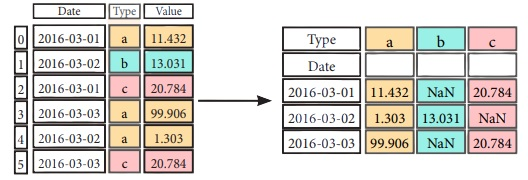Pivot Table

 df4 = pd.pivot_table(df2, #Spread rows into
columns values='Value', index='Date', columns='Type'])


Stack / Unstack

stacked= df5.stack() #Pivot o level of column labels
stacked.unstack() #Pivot o level of index labels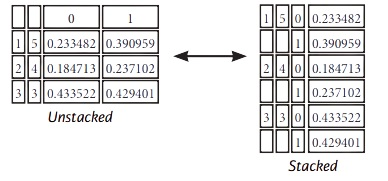Melt

 pd.melt(df2, #Gather columns into rows
id _vars=[°Date°],
value_var s=['Type','Value'],
value name=''Observations'')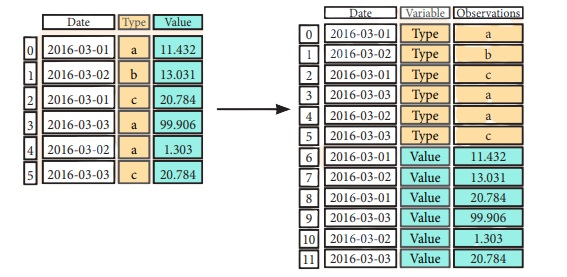### Iteration

 df.iteritems() #{Column-index, Series) pairs
df.iterrows() #{Row-index, Series) pairs



### Missing Data

 df.dropna() #Drop NaN values
df3.fillna(df3.mean()) #Fill NaN values with o predetermined value
df2.replace("a" , "f") #Replace values with others



Selecting

 df3.loc[:,(df3>1).any()] #Select cols with any vols >1
df3.loc[:,(df3>1).all()] #Select cols with vols> 1
df3.loc[:,df3.isnull().any()] #Select cols with NaN
df3.loc[:,df3.notnull().all()] #Select cols without NaN


Indexing With isin ()

 df[(df.Country.isin(df2.Type))] #Find some elements
df3.filter(iterns="a","b"]) #Filter on values
df.select(lambda x: not x%5) #Select specific elements


Where

 s.where(s > 0) #Subset the data


Query

 df6.query('second > first') #Query DataFrame


Setting/Resetting Index

 df.set_index('Country' ) #Set the index
df4 = df.reset_index() #Reset the index
df = df.rename(index=str, #Rename
DataFrame columns={	"Country":"cntry",
"Capital':"cptl",
"Population":pplt})


Reindexing

s2=s.reindex(['a','c','d','e','b'])


Forward Filling

 df.reindex(	range(4),
method= 'ffill')

	Country	Capital		Population
0	Belgium	Brussels	11190846
1	India	New Delhi	1303171035
2	Brazil	Brasilia	207847528
3	Brazil	Brasilia	207847528


Backward Filling

 s3 = s.reindex(	range(5),
method= 'bfill')

0	3
1	3
2	3
3	3
4	3


Multilndexing

arrays= [np.array([l,2,3]),
np.array([5,4,3])]
df5 = pd.DataFrame(np.random.rand(3, 2), index=arrays)
tuples= list(zip(*arrays))
index= pd.Multilndex.from_tuples(tuples,
names= [ 'first' , 'second' ])
df6 = pd.DataFrame(np.random.rand(3, 2), index=index)
df2.set_index([ "Date", "Type"])


### Duplicate Data

 s3.unique() #Return unique values
df2.duplicated('Type') #Check duplicates
df2.drop_dup licates( 'Type', keep='last') #Drop duplicates
df.index.duplicated() #Check index duplicates


### Grouping Data

Aggregation

 df2.groupby(by=['Date','Type']).mean()
df4.groupby(level=0).sum()
df4.groupby(level=0).agg({ 'a':lambda x:sum(x)/len (x), 'b': np.sum})


Transformation

 customSum = lambda x: (x+x%2)
df4.groupby(level=0).transform(customSum)


## Combining Data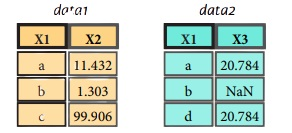Merge

 pd.merge(data1,
data2,
how = 'left' ,
on='X1')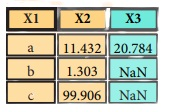pd.merge(data1,
data2,
how = 'right' ,
on='X1')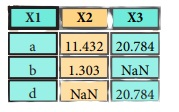pd.merge(data1,
data2,
how='inner',
on='X1')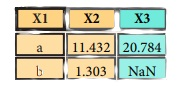pd.merge(data1,
data2,
how='outer',
on='X1')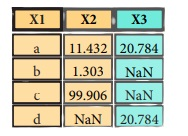Join

 datal.join(data2, ho w='righ t')


Concatenate

Vertical

 s.append(s2)


Horizontal/Vertical

 pd.concat([s,s2],axis=l, keys=['One' ,'Two'])
pd.concat([data1, data2], axis=l, join='inner')


### Dates

 df2['Date']= pd.to_da tetime(d f2['Date'])
df2['Date']= pd.da te_range( '2000-1-1',
periods=6, freq='M' )
dates= [datetime(2012,5,1), datetime(2012,5,2)]
index= pd.Datetimelndex(dates)
index= pd.date_range(datetime(2012,2,1), end, freq='BM' )



### Visualization

 import matplotlib.pyplot as plt
s.plot()
plt.show()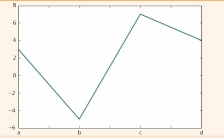df2.plot()
plt.show()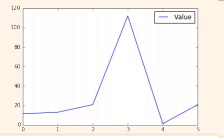# Data Wrangling with PandasThe Pandas library is built on NumPy and provides easy-to-use data structures and data analysis tools for the Python programming language.

Use the following import convention:

 import pandas as pd


### Pandas Data Structure

Series

A one-dimensional labeled array

capable of holding any data type

  s = pd.Series([3,-5,7, s], index=['a','b','c','d'])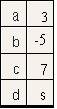Dataframe

A two-dimensional labeled data structure with columns of potentially different types

 data = { 'Country' : ['Belgium' ,'India ',' Brazil'  ] ,
'Capital': ['Brussels','New Delhi','Brasilia'] ,
'Population': [111908s6, 1303171035, 207847528]}
df = pd.DataFrame(data,columns=[ 'Country' , 'Capital' , 'Population' ])



Dropping

 s.drop(['a', 'c']) #Drop values from rows (axis=B)
df.drop( 'Country', axis=l) #Drop values from columns(axis=l)


Sort & Rank

 df.sort_index() #Sort by labels along an axis
df.sort_values( by='Country') #Sort by the values along on axis
df.rank() #Assign ranks to entries


### I/O

 pd.read_csv('file.csv', header=None, nrows=5)
df.to_csv('myDataFrame.csv')


 pd.read_excel( 'file.xlsx')
df.to_excel('dir/myDataFrame.x lsx', sheet_name= 'Sheet1')


Read multiple sheets from the same file

 xlsx = pd.ExcelFile('file.xls')


Read and Write to SQL Query or Database Table

 from sqlalchemy import create_engine
engine = create_eng ine('sqlite:///:memory:' )
pd.read_sql( "SELECT* FROM my_tabl e;", engine)
pd.read_sql_ tabl e('my_ tabl e', engine)
pd.read_sql_query( "SELECT * FROM my_table;", engine)



 df.to_sql('myDf',engine)


### Selection

Getting

 s['b'] #Get one element
-5

 df[l:] #Get subset of a DataFrome
Country Capital Population 1 India New Delhi 1303171035
2 Brazil Brasilia 207847528


Selecting, Boolean Indexing & Setting

By Position

 df.iloc[,] #Select single value by row & column
'Belgium'
df.iat(,)
'Belgium'


By Label

 df.loc[, [ 'Country']] #Select single value by row & column labels 'Belgium'
df.at(, [ 'Country ']) 'Belgium'


By Label/Position

 df.ix #Select single row of subset of rows
Country Brazil Capital Brasilia Population 207847528
df.ix[:,'Capital'] #Select a single column of subset of columns
0	Brussels
1	New Delhi
2	Brasilia
df.ix[1,'Capital'] #Select rows and columns 'New Delhi'


Boolean Indexing

 s[N(s > 1)] #Series s where value is not >l
s[(s < -1) I (s > 2)] #s where value is f-1 or >2
df[df['Population']>1200000000] #Use filter to adjust DataFrame


Setting

 s['a'] = 6 #Set index a of Series s to 6


### Retrieving Series/DataFrame Information

Basic Information

 df.shape #(rows,columns)
df.index #Describe index
df.columns #Describe DataFrame columns
df.info() #Info on DataFrame
df.count() #Number of non-NA values


Summary

 df.sum() #Sum of values
df.cumsum() #Cummulative sum of values
df.min() /df.max() #11inimum/maximum values
df.idxmin()/df.idxmax() #Minimum/Maximum index value
df.describe() #Summary statistics
df.mean() #11ean of values
df.median() #Median of values


### Applying Functions

 f = lambda x: X*2
df.apply(f) #Apply function
df.applymap(f) #Apply function element-wise


Data Alignment

Internal Data Alignment

 s3 = pd.Series([7, -2, 31, index= ['a','c' ,'d'])
s + s3
a 10.0
b NaN
C 5.0
d 7.0


Arithmetic Operations with Fill Methods

You can also do the internal data alignment yourself with the help of the fill methods:

 s.add(s3, fill_values=0) a 10.0
b -5. 0
C 5.0
d 7.0
s.sub(s3, fill_value=2)
s.div(s3, fill_value=4)
s.mul(s3, fill_value=3)


# Section 4

## Data Analysis with NumpyThe NumPy library is the core library for scientific computing in Python. It provides a high performance multidimensional array object , and tools for working with these arrays

Use the following import convention

import numpy as np


Creating Array

 a = np.array([l,2,3])
b = np.array([(l.5,2,3), (4,5,6)], dtype = float)
c = np.array([[(l.5,2,3), (4,5,6)],[(3,2,1), (4,5,6)]], dtype = float)


Initial Placeholders

 np.zeros((3,4)) #Create an array af zeros
np.ones((2,3,4),dtype=np.int16) #Create an array of ones
d = np.arange(10,25,5) #Create an array of evenly spaced values (step value)
np.linspace(0,2,9) #Create an array of evenly spaced values (number of samples)
e = np.full((2,2),7) #Create a constant array
f = np.eye(2) #Create a 2X2 identity matrix
np.random.random((2,2)) #Create an array with random values
np.empty((3,2)) #Create an empty array



### I/O

 np.save('my_array',a)
np.save('array.npz',a, b)


 np.loadtxt("myfile.txt")
np.genfromtxt("my_file.csv", delimiter=',' )
np.savetxt("myarray.txt" , a, delimiter=" " )


 a.shape #Array dimensions
len(a) #Length of array
b.ndim #Number of array dimensions
e.size #Number of array elements
b.dtype #Data type of array elements
b.dtype.name #Name of data type
b.astype(int) #Convert an array to a different type


Data Type

 np.int64 #Signed 64-bit integer types
np.flaat32 #Standard double-precision floating paint
np.complex #Complex numbers represented by 128 floats
np.baol #Boolean type storing TRUE and FALSE values
np.object #Python object type
np.string _ #Fixed-length string type
np.unicode_ #Fixed-length unicode type


## Array Mathematics

Arithmetic Operations

 g = a - b #Subtraction
array([[-0.5,0. , 0. ],
[-3. , -3. , -3. ]])
np.subtract(a,b) #Subtraction
array([[ 2.5, 4. , 6. ],
[5.,7.,9.]])
a / b #Division
array([[ 0.66666667, 1., 1.],
[ 0.25 , 0.4 , 0.5 ]])
np.divide(a,b) #Division
a * b #Multiplication
array([[ 1.5, 4. , 9. ],
[ 4. , 10. , 18. ]])
np.multiply(a,b) #Multiplication
np.exp(b) #Exponentiation
np.sqrt(b) #Square root
np.sin(a) #Print sines of an array
np.cos(b) #Element-wise cosine
np.log(a) #Element-wise natural logarithm
e.dot(f) #Dot product
array([[ 7., 7.],


Comparison

a == b #Element-wise comparison
array([[False, True , True],
[ False, False, False]], dtype=bool)
a < 2 #Element-wise comparison
array([True , False, False], dtype=bool)
np.array_equal(a, b) #Array-wise comparison


Aggregate Functions

 a.sum() #Array-wise sum
a.min() #Array-wise minimum value
b.max(axis=0) #Maximum value of an array row
b.cumsum(axis=l) #Cumulative sum of the elements
a.mean() #Mean
b.median() #Median
a.corrcaef() #Correlation coefficient
np.std(b) #Standard deviation


### Copying Array

 h = a.view() #Create a view af the array with the some data
np.capy(a) #Create a copy of the array
h = a.copy() #Create a deep copy of the array


### Sorting Array

Subsetting

 a #Select the element at the 2nd index
3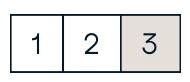b[1,2] #Select the element at row 1 column 2 (equivalent to b)
6.0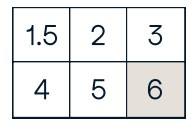Slicing

 a[0:2] #Select items at index 0 and 1
array([1, 2])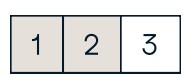b[0:2,1] #Select items at rows 0 and 1 in column 1
array([ 2., 5.])

 b[:1] #Select all items at row 0 (equivalent to b[0:1, :])
array([[1.5, 2., 3.]])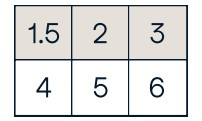c[1,... ] #Same as [1,:,:]
array([[[ 3., 2., 1.],
[  4., 5.,	6.]]])
a[: :-1] #Reversed array a array([3, 2, 1])


Boolean Indexing

 a[a<2] #Select elements from a less than 2
array()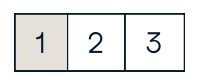Fancy Indexing

 b[[l, 0, 1, 0],[0, 1, 2, 0]] #Select elements (1,0),(0,1),(1,2) and (0,0)
array([ 4. , 2. , 6. , 1.5])
b[[l, 0, 1, 0]][:,[0,1,2,0]] #Select a subset of the matrix's rows and columns array([[ 4. ,5. , 6. , 4. ],
[ 1.5, 2.3,	1.5],


### Array Manipulation

Transposing Array

 i = np.transpose(b) #Permute array dimensions
i.T #Permute array dimensions


Changing Array Shape

 b.ravel() #Flatten the array
g.reshape(3,-2) #Reshape, but don't change data


 h.resize((2,6)) #Return a new array with shape (2,6)
np.append(h,g) #Append items to an array
np.insert(a, 1, 5) #Insert items in an array
np.delete(a,) #Delete items from an array


Combining Arrays

 np.concatenate((a,d),axis=0) #Concatenate arrays array([ 1, 2, 3, 10, 15, 20])
np.vstack((a,b)) #Stack arrays vertically (row-wise)
array([[ 1. , 2.	3. ],
[ 1.5, 2. , 3. ],
[   4.    ,   5.    ,   6.    ]])
np.r_[e,f] #Stack arrays vertically (row -wise)
np.hstack((e,f)) #Stack arrays horizontally (col umn-wise)
array([[ 7., 7., 1., 0.],
[ 7., 7., 0., 1.]])
np.column_stack((a,d)) #Create stacked column-wise arrays
array([[ 1, 10],
[ 2,  15],
[ 3, 20]])
np.c_[a ,d] #Create stacked column-wise arrays



Splitting Arrays

 np.hsplit(a,3) #Split the array horizontally at the 3rd index
[array(),array(),array()]
np.vsplit(c,2) #Split the array vertically at the 2nd index
[array([[[ 1.5, 2. , 1. ],
[ 4. , 5. , 6. ]]]),
array([[[ 3., 2., 3.],
[  4.,	5.,	6.]]])]


# Section 5

## Data Visualization with Matplotlib

Matplotlib is a Python 2D plotting library which produces publication-quality figures in a variety of hardcopy formats and interactive environments across platforms.

1D Data

 import numpy as np
X = np.linspace(0, 10, 100)
y = np.cos(x)
z = np.sin(x)



2D Data or Images

 data= 2 * np.random.random((10, 10))
data2 = 3 * np.random.random((10, 10))
Y, X = np.mgrid[-3:3:100j, -3:3:100j]
U = -1 - X**2 + Y
V = 1 +  X - Y**2
from matplotlib.cbook import get_sample_data
img = np.load(g et_sample_data('axes_gr id/bivar iate_normal.npy '))


### Create Plot

import matplotlib.pyplot as plt


Figure

 fig = plt.figure()
fig2 = plt.figure(figsize=plt.figaspect(2.0))


Axes

ll plotting is done with respect to an Axes. In most cases, a subplot will fit your needs. A subplot is an axes on a grid system.

 fig.add_axes()
fig3, axes= plt.subplots(nrows=2,ncols=2)
fig4, axes2 = plt.subplots(ncols=3)


Save Plot

 plt.savefig( 'foe.png' J #Save figures
plt.savefig( 'foo.png',transparent=True) #Save transparent figures


Show Plot

 plt.show()


### Plotting Routines

1D Data

 fig, ax= plt.subp lots()
lines= ax.plot(x,y) #Draw points with lines or markers connecting them
ax.scatter(x,y) #Draw unconnected points, scaled or colored
axes[0,0].bar([l,2,3],[3,4,5]) #Plot vertical rectangles (constant width)
axes[l,0].barh([0.5,1,2.5],[0,1,2]) #Plot horiontol rectangles (constant height)
axes[l,1].axhline(0.s5) #Draw a horizontal line across axes
axes[0,1].axvline(0.65) #Draw a vertical line across axes
ax.fill(x,y,color='blue') #Drow filled polygons
ax.fill_between(x,y,color='yellow') #Fill between y-values and 0



2D Data

 fig, ax= plt.subplots()
im = ax.imshow(img, #Colormapped or RGB arrays
cmap= 1 gist_ear th 1 ,
interpolation= 1 neare st',
vmin=-2,
vmax =2)
axes2.pcolor(data2) #Pseudocolor plot of 2D array
axes2.pcolormesh(data) #Pseudocolor plot of 2D array
CS= plt.contour(Y,X,U) #Plot contours
axes2.contourf(datal) #Plot filled contours
axes2= ax.clabel(CS) #Lobel a contour plot



Vector Fields

 axes[0,1].arrow(0,0,0.5,0.5) #Add an arrow to the axes
axes[l,l].quiver(y,z) #Plot a 2D field of arrows
axes[0,1].streamplot(X,Y,U,V) #Plot a 2D field of arrows



Data Distributions

 axl.hist(y) #Plot a histogram
ax3.boxplot(y) #Make a box and whisker plot
ax3.violinplot(z) #Make a violin plot



### Plot Anatomy

The basic steps to creating plots with matplotlib are:

1 Prepare Data 2 Create Plot 3 Plot 4 Customized Plot 5 Save Plot 6 Show Plot

 import matplotlib.pyplot as plt
x = [1,2,3,s] #Step 1
y = [ 10,20,25,30]
fig= plt.figure() #Step 2
ax.plot(x, y, color='lightb lue', linewidth=3) #Step 3, 4
ax.scatter([2,4,6],
[5,15,25],
color='darkgreen ',
marker='^')
ax.set_xlim(l, 6.5)
plt.savefig('foo.png' ) #Step 5
plt.show() #Step 6


Close and Clear

 plt.cla() #Clear on axis
plt.clf() #Clear the entire figure
plt.close() #Close a window


### Plotting Cutomize Plot

Colors, Color Bars & Color Map

 plt.plot(x, x, x, X**2, x, X**3)
ax.plot(x, y, alpha = 0.s)
ax.plot(x, y, c='k' )
fig.colorbar(im, orientation= 'horizontal')
im = ax.imshow(img,cmap=  'seismic'  )



Markers

 fig, ax= plt.subplots()
ax.scatter(x,y,marker="."   )
ax.plot(x,y ,marker="o")


Linestyles

 plt.plot(x,y,linewidth=4.0)
plt.plot(x,y,ls='solid')
plt.plot(x,y,ls='--' )
plt.plot(x, y,'-- 1 ,X**2,Y** 2, '-.')
plt.setp(lines,color='r',linewidth= 4.0)


Text & Annotations

 ax.text(1,-2.1,'Example Graph' , style='italic')
ax.annotate("Sine",
xy=(8, 0),
xycoords= 'data',
xytext=(10.5, 0),
textcoords= 1 data',
arrowprops=dict(arrowstyle= "->" connectionstyle="arc3"),)


Mathtext

 plt.title(r'$sigma_ i=15$', fontsize=20)


Limits, Legends and Layouts

Limits & Autoscaling

 ax.margins(x=0.0,y=0.1) #Add padding to a plot
ax.axis('equa l') #Set the aspect ratio of the plot to 1
ax.set(xlim=[0,10.5],ylim=[-1.5,l.5]) #Set limits for x-and y-axis
ax.set_xlim(0,10.5) #Set limits for x-axis


Legends

 ax.set(	title='An Example Axe s', #Set a title and x-ond y-axis labels
ylabel='Y-Axis',
xlabel='X-Axis')
ax.legend( loc='best') #No overlapping plot elements


Ticks

 ax.xaxis.set(ticks=range(l,5), #Manually set x-ticks
ticklabels=[3,100,-12,"foo']')
#Makey-ticks longer and go in and out
ax.tick_param s(axis='y',direction='inout ',length=10)



Subplot Spacing

 fig3.subplots_ad just(wspace=0.5, #Adjust the spacing between subplots
hspace=0.3, left=0.125, right=0.9, top=0.9, bottom=0.1)
fig.tight_layout() #Fit subplot(s) in to the figure area


Axis Spines

 axl.spines['top'].set_visible(False)
#Make the top axis line for a plot invisible
axl.spines['bottom'].set_po sition(('outward' ,10))
#Move the bottom axis line outward


# Queries in SQL## Querying data from a table

Query data in columns c1, c2 from a table

SELECT c1, c2 FROM t;


Query all rows and columns from a table

SELECT * FROM t;


Query data and filter rows with a condition

SELECT c1, c2 FROM t
WHERE condition;


Query distinct rows from a table

SELECT DISTINCT c1 FROM t
WHERE condition;


Sort the result set in ascending or descending order

SELECT c1, c2 FROM t
ORDER BY c1 ASC [DESC];


Skip offset of rows and return the next n rows

SELECT c1, c2 FROM t
ORDER BY c1
LIMIT n OFFSET offset;


Group rows using an aggregate function

SELECT c1, aggregate(c2)
FROM t
GROUP BY c1;


Filter groups using HAVING clause

SELECT c1, aggregate(c2)
FROM t
GROUP BY c1
HAVING condition;


## Querying from multiple tables

Inner join t1 and t2

SELECT c1, c2
FROM t1
INNER JOIN t2 ON condition;


Left join t1 and t1

SELECT c1, c2
FROM t1
LEFT JOIN t2 ON condition;


Right join t1 and t2

SELECT c1, c2
FROM t1
RIGHT JOIN t2 ON condition;


Perform full outer join

SELECT c1, c2
FROM t1
FULL OUTER JOIN t2 ON condition;


Produce a Cartesian product of rows in tables

SELECT c1, c2
FROM t1
CROSS JOIN t2;


Another way to perform cross join

SELECT c1, c2
FROM t1, t2;


Join t1 to itself using INNER JOIN clause

SELECT c1, c2
FROM t1 A
INNER JOIN t1 B ON condition;


## Using SQL Operators

Combine rows from two queries

SELECT c1, c2 FROM t1
UNION [ALL]
SELECT c1, c2 FROM t2;


Return the intersection of two queries

SELECT c1, c2 FROM t1
INTERSECT
SELECT c1, c2 FROM t2;


Subtract a result set from another result set

SELECT c1, c2 FROM t1
MINUS
SELECT c1, c2 FROM t2;


Query rows using pattern matching %, _

SELECT c1, c2 FROM t1
WHERE c1 [NOT] LIKE pattern;


Query rows in a list

SELECT c1, c2 FROM t
WHERE c1 [NOT] IN value_list;


Query rows between two values

SELECT c1, c2 FROM t
WHERE  c1 BETWEEN low AND high;


Check if values in a table is NULL or not

SELECT c1, c2 FROM t
WHERE  c1 IS [NOT] NULL;


## Managing tables

Create a new table with three columns

CREATE TABLE t (
id INT PRIMARY KEY,
name VARCHAR NOT NULL,
price INT DEFAULT 0
);


Delete the table from the database

DROP TABLE t ;


Add a new column to the table

ALTER TABLE t ADD column;


Drop column c from the table

ALTER TABLE t DROP COLUMN c ;


ALTER TABLE t ADD constraint;


Drop a constraint

ALTER TABLE t DROP constraint;


Rename a table from t1 to t2

ALTER TABLE t1 RENAME TO t2;


Rename column c1 to c2

ALTER TABLE t1 RENAME c1 TO c2 ;


Remove all data in a table

TRUNCATE TABLE t;


## UsingSQL constraints

Set c1 and c2 as a primary key

CREATE TABLE t(
c1 INT, c2 INT, c3 VARCHAR,
PRIMARY KEY (c1,c2)
);


Set c2 column as a foreign key

CREATE TABLE t1(
c1 INT PRIMARY KEY,
c2 INT,
FOREIGN KEY (c2) REFERENCES t2(c2)
);


Make the values in c1 and c2 unique

CREATE TABLE t(
c1 INT, c1 INT,
UNIQUE(c2,c3)
);


Ensure c1 > 0 and values in c1 >= c2

CREATE TABLE t(
c1 INT, c2 INT,
CHECK(c1> 0 AND c1 >= c2)
);


Set values in c2 column not NULL

CREATE TABLE t(
c1 INT PRIMARY KEY,
c2 VARCHAR NOT NULL
);


## Modifying Data

Insert one row into a table

INSERT INTO t(column_list)
VALUES(value_list);


Insert multiple rows into a table

INSERT INTO t(column_list)
VALUES (value_list),
(value_list), …;


Insert rows from t2 into t1

INSERT INTO t1(column_list)
SELECT column_list
FROM t2;


Update new value in the column c1 for all rows

UPDATE t
SET c1 = new_value;


Update values in the column c1, c2 that match the condition

UPDATE t
SET c1 = new_value,
c2 = new_value
WHERE condition;


Delete all data in a table

DELETE FROM t;


Delete subset of rows in a table

DELETE FROM t
WHERE condition;


## Managing Views

Create a new view that consists of c1 and c2

CREATE VIEW v(c1,c2)
AS
SELECT c1, c2
FROM t;


Create a new view with check option

CREATE VIEW v(c1,c2)
AS
SELECT c1, c2
FROM t;
WITH [CASCADED | LOCAL] CHECK OPTION;


Create a recursive view

CREATE RECURSIVE VIEW v
AS
select-statement -- anchor part
UNION [ALL]
select-statement; -- recursive part


Create a temporary view

CREATE TEMPORARY VIEW v
AS
SELECT c1, c2
FROM t;


Delete a view

DROP VIEW view_name;



## Managing indexes

Create an index on c1 and c2 of the t table

CREATE INDEX idx_name
ON t(c1,c2);



Create a unique index on c3, c4 of the t table

CREATE UNIQUE INDEX idx_name
ON t(c3,c4)



Drop an index

DROP INDEX idx_name;



## Managing triggers

Create or modify a trigger

CREATE OR MODIFY TRIGGER trigger_name
WHEN EVENT
ON table_name TRIGGER_TYPE
EXECUTE stored_procedure;



WHEN

• BEFORE – invoke before the event occurs
• AFTER – invoke after the event occurs

EVENT

• INSERT – invoke for INSERT
• UPDATE – invoke for UPDATE
• DELETE – invoke for DELETE

TRIGGER_TYPE

• FOR EACH ROW
• FOR EACH STATEMENT

Delete a specific trigger

DROP TRIGGER trigger_name;



# Machine Learning with Scikit-LearnScikit-learn is an open source Python library that implements a range of machine learning, preprocessing, cross-validation and visualization algorithms using a unified interface.

Example

 from sklearn import neighbors, datasets, preprocessing
from sklearn.model_selection import train_test_split
from sklearn.metrics import accuracy_score
X, y = iris.data[:, :2], iris.target
X_train, X_test, y_train, y_test = train_test_split(X, y, random_state=33)
scaler= preprocessing.StandardScaler().fit(X_train)
X_train = scaler.transform(X_train)
X_test = scaler.transform(X_test)
knn = neighbors.KNeighborsClassifier(n_neighbors=5)
knn.fit(X_train, y_train)
y_pred = knn.predict(X_test)
accuracy_score(y _test, y_pred)


Your data needs to be numeric and stored as NumPy arrays or SciPy sparse matrices. Other types that are convertible to numeric arrays, such as Pandas DataFrame, are also acceptable

 import numpy as np
X = np.random.random((10,5))
Y = np. array (['M', 'M' ,'F','F','M','F','M','M','F','F','F'])
X[X < 0.7] = 0


### Training And Test Data

 from sklearn.model_selection import train_test_split
X_train, X_test, y_train, y_test = train_test_split(X,y, random_state=0)



### Model Fitting

Supervised learning

 lr.fit(X, y) #Fit the model to the data
knn.fit(X_train, y_train)
svc.fit(X_train, y_train)


Unsupervised Learning

 k_mean s.fit(X_train) #Fit the model to the data
pca_model = pca.fit_transform(X_train) #Fit to data, then transform it


### Prediction

Supervised Estimators

 y_pred = svc.predict(np.random.random((2,5))) #Predict labels
y_pred = lr.predict(X_test) #Predict labels
y_ pred = knn.predict_proba(X_test) #Estimate probability of a label


Unsupervised Estimators

 y_pred = k_means.predict(X_test) #Predict labels in clustering algos


### Preprocessing The Data

Standardization

 from sklearn.preprocessing import StandardScaler
scaler= StandardScaler().fit(X_train)
standardized_X = scaler.transform(X_train)
standardized_X_test= scaler.transform(X _test)


Normalization

 from sklearn.preprocessing import Normalizer
scaler= Normalizer().fit(X_train)
normalized_X = scaler.transform(X _train)
normalized_X_test = scaler.transform(X_test)


Binarization

 from sklearn.preprocessing import Binarizer
binarizer = Binarizer(threshold=0.0).fit(X)
binary_X = binarizer.transform(X)



Encoding Categorical Features

 from sklearn.preprocessing import LabelEncoder
enc= LabelEncoder()
y = enc.fit_transform(y)


Imputing Missing Values

 from sklearn.preprocessing import Imputer
imp= Imputer(missing_values=0, strategy='mean ', axis=0)
imp.fit_transform(X_train)


Generating Polynomial Features

 from sklearn.preprocessing import PolynomialFeatures
poly= PolynomialFeatures(5)
poly.fit_transform(X)


### Supervised Learning Estimators

Linear Regression

 from sklearn. linear m_ odel import LinearRegression
lr = LinearRegression(normalize=True)


Support Vector Machines (SVM)

 from sklearn.svm import SVC
SVC= SVC(kernel='linear')


Naive Bayes

 from sklearn.naive_bayes import GaussianNB
gnb = GaussianNB()


KNN

 from sklearn import neighbors
knn = neighbors.KNeighborsClassifier(n_neighbors=5)


### Unsupervised Learning Estimators

Principal Component Analysis (PCA)

 from sklearn.decomposition import PCA
pea= PCA(n_components=0.95)


K Means

 from sklearn.cluster import KMeans
k_means = KMeans(n_clusters=3, random_state=0)


### Classification Metrics

Accuracy Score

 knn.score(X_test, y_test) #Estimator score method
from sklearn.metrics import accuracy_score #Metric scoring functions
accuracy_score(y_test, y_pred)


Classification Report

 from sklearn.metrics import classification_report #Precision, recall, fl-score and support
print(classification_report(y_test, y_pred))


Confusion Matrix

 from sklearn.metrics import confusion_matrix
print(confusion_matrix(y_test.y_pred))


### Regression Metrics

Mean Absolute Error

 from sklearn.metrics import mean_absolute_error
y_true = [3, -0.5,2]
mean_absolute_error(y_true, y_pred)



Mean Squared Error

 from sklearn.metrics import mean_squared_error
mean_squared _error(y_test, y_ pred)


R2 Score

 from sklearn.metrics import r2_score
r2_score(y_true, y_ pred)


### Clustering Metrics

 from sklearn.metrics import adjusted_rand_score



Homogeneity

 from sklearn.metrics import homogeneity_score
homogeneity_score(y_true, y_pred)


V-measure

 from sklearn.metrics import v_measure_score
metrics.v_measure_score(y_true , y_pred)


### Cross-Validation

 from sklearn.cross_validation import cross_val_score
print(cross_val_score(knn, X_train, y_train, cv=4))
print(cross_val_score(lr, X, y, cv=2))


 from sklearn.grid_search import GridSearchCV
params = {"n_neighbors "  : np.arange(l,3),
"metric "	 : [ "euclidean" , "cityblock "] }
grid= GridSearchCV(estimator=knn,
param_grid=params)
grid.fit(X_train, y_train)
print(grid.best_score_)
print(grid.best_estimator_.n_neighbors)


Randomized Parameter Optimization

 from sklearn.grid _search import RandomizedSearchCV
params = { "n_neighbors":range(l,5), "weights": ["unifomr","distance"]}
rsearch = RandomizedSearchCV(estimator=knn, param_distributions=params,
cv=4, n_iter=S, random_state=5)
rsearch.fit(X_train, y_train)
print(rsearch.best_score_)


# Section 8

## SciPyThe SciPy library is one of the core packages for scientific computing that provides mathematical algorithms and convenience functions built on the NumPy extension of Python.

 import numpy as np
a= np.array([1,2,3])
b = np.array([(1+5j,2j,3j), (4j,5j,6j)])
c = np.array([[(1.5,2,3), (4,5,6)], [(3,2,1), (4,5,6)]])


Index Tricks

 np.mgrid[0:5,0:5] #Create a dense meshgrid
np.ogrid[0:2,0:2] #Create an open meshgrid
np.r_[[3,*5,-1:1:10j] #Stack arrays vertically (row-wise)
np.c_[b,c] #Create stocked column-wise arrays


Shape Manipulation

 np.transpose(b) #Permute array dimensions
b.flatten() #Flatten the array
np.hstack((b,c)) #Stack arrays horizontally (column-wise)
np.vstack((a,b)) #Stack arrays vertically (row-wise)
np.hsplit(c,2) #Split the array horizontally at the 2nd index
np.vpslit(d,2) #Split the array vertically at the 2nd index


Polynomials

 from numpy import polyld
p = poly1d([3,4,5]) #Create a polynomial object


Vectorizing Functions

 def myfunc(a): if a< 0:
return a*2
else:
return a/2
np.vectorize(myfunc) #Vectorize functions



Type Handling

 np.real(c) #Return the real part of the array elements
np.imag(c) #Return the imaginary part of the array elements
np.real_if_close(c,tol=1000) #Return a real array if complex parts close to 0
np.cast['f'](np.pi) #Cast object to a data type


Other Useful Functions

 np.angle(b,d eg=True) #Return the angle of the complex argument
g = np.linspace(0,np.pi,num=5) #Create an array of evenly spaced values(number of samples)
g [3:] += np.pi
np.unwrap(g) #Unwrap
np.logspace(0,10,3) #Create an array of evenly spaced values (log scale)
np.select([c<li],[c*2]) #Return values from a list of arrays depending on conditions
misc.factorial(a) #Factorial
misc.comb( 10,3,exact=True) #Combine N things taken at k time
misc.central_diff_weights(3) #Weights for Np-point central derivative
misc.derivative(myfunc,1.0) #Find then-th derivative of a function at a point



### Linear Algebra

You’ll use the linalg and sparse modules. Note that scipy. linalg contains and expands on numpy. linalg.

 from scipy import linalg, sparse


Creating Matrices

 A = np.matrix(np.random.random((2,2)))
B = np.asmatrix(b)
C = np.mat(np.random.random((10,5)))
D = n p.mat([[3,Ii],  [5,6]])


Basic Matrix Routines

 A.I #Inverse
linalg.inv(A)  #Inverse
A.T #Tranpose matrix
A.H #Conjugate transposition
np.trace(A) #Trace


Norm

 linalg.norm(A) #Frobenius norm
linalg.norm(A,1) #Ll norm (max column sum)
linalg.norm(A,np.inf) #L inf norm (max row sum)


Rank

 np.linalg.matrix_rank(C) #Matrix rank


Determinant

 linalg.det(A) #Determinant


Solving linear problems

 linalg.solve(A,b) #Solver for dense matrices
E = np.mat(a).T #Solver for dense matrices
linalg.lstsq(D,E) #Le ast-squares solution to linear matrix equation


Generalized inverse

 linalg.pinv(C) #Compute the pseudo-inverse of a matrix (least-squares solver)
linalg. pinv2(C) #Compute the pseudo-inverse of a matrix (SVD)


### Creating Sparse Matrices

 F = np.eye(3, k=l) #Create a 2X2 identity matrix
G = np.mat(np.identity(2)) #Create a 2x2 identity matrix
C[C > 0.5] = 0
H = sparse.csr_matrix(C) #Compressed Sparse Row matrix
I=	sparse.csc_matrix(D) #Compressed Sparse Column matrix
J = sparse.dok_matrix(A) #Dictionary Of Keys matrix
E.tadense() #Sparse matrix to full matrix
sparse.isspmatrix_csc(A)


Sparse Matrix Routines

Inverse

 sparse.linalg.inv(I) #Inverse


Norm

 sparse.linalg.norm(I) #Norm


Solving linear problems

 sparse.linalg.spsolve(H,I) #Solver for sparse matrices


Sparse Matrix Functions

 sparse.linalg.expm(I) #Sparse matrix exponential


Sparse Matrix Decompositions

 la, v = sparse.linalg.eigs(F,1) #Eigenvalues and eigenvectors
sparse.linalg.svds(H, 2) #SVD


## Matrix Function

 np.add(A,D) #Addition


Subtraction

 np.subtract(A,D) #Subtraction


Division

 np.divide(A,D) #Division


Multiplication

 np.multiply(D,A) #Multiplication
np.dot(A,D) #Dot product
np.vdot(A,D) #Vector dot product
np.inner(A,D) #Inner product
np.outer(A,D) #Outer product
np.tensardat(A,D) #Tensor dot product
np.kron(A,D) #Kronecker product


Exponential Functions

 linalg.expm(A) #Matrix exponential
linalg.expm2(A) #Matrix exponential (Taylor Series)
linalg.expm3(D) #Matrix exponential (eigenvalue decomposition)


Logarithm Function

 linalg.lagm(A) #Matrix logarithm


Trigonometric Functions

 linalg.sinm(D) Matrix sine
linalg.cosm(D) Matrix cosine
linalg.tanm(A) Matrix tangent


Hyperbolic Trigonometric Functions

 linalg.sinhm(D) #Hypberbolic matrix sine
linalg.coshm(D) #Hyperbolic matrix cosine
linalg.tanhm(A) #Hyperbolic matrix tangent


Matrix Sign Function

 np.sigm(A) #Matrix sign function


Matrix Square Root

 linalg.sqrtm(A) #Matrix square root


Arbitrary Functions

 linalg.funm(A, lambda x: X*X) #Evaluate matrix function


Eigenvalues and Eigenvectors

 la, v = linalg.eig(A) #Solve ordinary or generalized eigenvalue problem for square matrix
l1, l2 = la #Unpack eigenvalues
v[:,0] #First eigenvector
v[:,1] #Second eigenvector
linalg.eigvals(A) #Unpack eigenvalues


Singular Value Decomposition

 U,s,Vh = linalg.svd(B) #Singular Value Decomposition (SVD)
M,N = B.shape
Sig= linalg.diagsvd(s,M,N) #Construct sigma matrix in SVD


LU Decomposition

 P,L,U = linalg.lu(C) #LU Decomposition


# Section 9

## Neural Networks with KerasKeras is a powerful and easy-to-use deep learning library for Theano and TensorFlow that provides a high-level neural networks API to develop and evaluate deep learning models.

A Basic Example

 import numpy as np
from keras.models import Sequential
from keras.layers import Dense
data= np.random.random((1000,100))
labels= np.random.randint(2,size=(1000,1))
model= Sequential()
activation='relu', input_dim=100))
model.compile(optimizer='rmsprop' ,
loss='binary_crossentropy',
metrics=['accuracy' ])
model.fit(data,labels,epochs=10,batch_size=32)
predictions= model.predict(data)


### Data

Your data needs to be stored as NumPy arrays or as a list of NumPy arrays. Ideally, you split the data in training and test sets, for which you can also resort to the train_test_split module of sklearn. cross_ validation.

Keras Data Sets

 from keras.datasets import boston_housing, mnist, cifar10, imdb
num_classes = 10


Other

 from urllib.request import urlopen
data =
X = data[:,0:8]
y = data [:,8]


### Preprocessing

 from keras.preprocessing import sequence


One-Hot Encoding

 from keras.utils import to_categorical
>>Y_train = to_categorical(y_train,num_classes)
Y_test = to_categorical(y_test,num_classes)
Y_train3 = to_categorical(y_train3,num_classes
Y_test3 = to_categorical(y_test3,num_classes)


### Model Architecture

Sequential Model

 from keras.models import Sequential
model= Sequential()
model2 = Sequential()
model3 = Sequential()


### Multilayer Perceptron (MLP)

Binary Classification

 from keras.layers import Dense
input _dim=8,
kernel_initializer='uniform',
activation='relu') )


Multi-Class Classification

 from keras.layers import Dropout



Regression

 model.add(Dense(64,activation='relu',input_dim=train_data.shape))


## Convolutional Neural Network (CNN)

 from keras.layers import Activation,Conv2D,MaxPooling20,Flatten



### Recurrent Neural Network (RNN)

 from keras.klayers import Embedding,LSTM


### Prediction

 model3.predict(x_test4, ba tch_size=32)
model3.predict_classes(x_test4,batch _size=32)


Train and Test Sets

 from sklearn.mode l_selection import train_test_split
X_train5,X_test5,y_train5,y_test5 = train_test_split(X, y,
test_size=0.33, random_state=42)


Standardization/Normalization

 from sklearn.preprocessing import StandardScaler
scaler= StandardScaler().fit(x_train2)
standa rdized_X = scaler.transform(x _train2)
standardized X test= scaler.transform(x_test2


### Inspect Model

 model.output_shape #Model output shape
model.summary() #Model summary representation
model.get_config() #Model configuration
model.get_weights()#List all weight tensors in the model


### Compile Model

MLP: Binary Classification

 model.compile(optimizer='adam' ,
loss= 'binary_crossentropy',
metrics=['accuracy'])


MLP: Multi-Class Classification

 model.compile(optimizer='rmsprop',
loss='categorical_crossentropy   ,
metrics=[ 'accuracy'])


MLP: Regression

 model.compile(optimizer='rmsprop',
loss= 'mse,' metrics=['mae'])


Recurrent Neural Network

model3.compile(loss='binary_crossentropy ',
metrics=['accuracy'])


Model Training

model3.fit(x_train4,
y_train4,
batch_size=32,
epochs=15,
verbose=1,
validation_data=(x_test4,y_test4))


score = model3.evaluate(x_test,
y_test,
batch_size=32)


 from keras.models import load_model
model3.save( )


Model Fine-tuning

Optimization Parameters

from keras.optimizers import RMSprop
opt = RMSprop(lr=0.0001, decay=1e-6)
model2.compile(loss= , 'categorical_crossentropy'
optimizer=opt,
metrics=[ 'accuracy'])


Early Stopping

 from keras.callbacks import EarlyStopping
early_stopping_monitor = EarlyStopping(patience=2)
model3.fit(x_train4,
y_train4,
batch_size=32,
epochs=15,
validation_data=(x_test4,y_test4),
callbacks=[early_stopping_monitor])


# PySpark & Spark SQLWhat is PySpark?

PySpark is an Apache Spark interface in Python. It is used for collaborating with Spark using APIs written in Python. It also supports Spark’s features like Spark DataFrame, Spark SQL, Spark Streaming, Spark MLlib and Spark Core.

What is PySpark SparkContext?

PySpark SparkContext is an initial entry point of the spark functionality. It also represents Spark Cluster Connection and can be used for creating the Spark RDDs (Resilient Distributed Datasets) and broadcasting the variables on the cluster.

 from pyspark.sql import SparkSession
spark = SparkSession \
.builder \
.appName( ) \
.config( , ) \
.getOrCreate()



What is spark-submit?

Spark-submit is a utility to run a pyspark application job by specifying options and configurations.

spark-submit \
--master <master-url> \
--deploy-mode <deploy-mode> \
--conf <key<=<value> \
--driver-memory <value>g \
--executor-memory <value>g \
--executor-cores <number of cores> \
--jars <comma separated dependencies> \
--packages <package name> \
--py-files \
<application> <application args>


where

–master : Cluster Manager (yarn, mesos, Kubernetes, local, local(k))
–deploy-mode: Either cluster or client
–conf: We can provide runtime configurations, shuffle parameters, application configurations using –conf. Ex: –conf spark.sql.shuffle.partitions = 300
–driver-memory : Amount of memory to allocate for a driver (Default: 1024M).
–executor-memory : Amount of memory to use for the executor process.
–executor cores : Number of CPU cores to use for the executor process.

What are RDDs in PySpark?

RDDs expand to Resilient Distributed Datasets. These are the elements that are used for running and operating on multiple nodes to perform parallel processing on a cluster. Since RDDs are suited for parallel processing, they are immutable elements. This means that once we create RDD, we cannot modify it. RDDs are also fault-tolerant which means that whenever failure happens, they can be recovered automatically. Multiple operations can be performed on RDDs to perform a certain task.

• Data Representation: RDD is a distributed collection of data elements without any schema
• Optimization: No in-built optimization engine for RDDs
• Schema: we need to define the schema manually.
• Aggregation Operation: RDD is slower than both Dataframes and Datasets to perform simple operations like grouping the data

Creation of RDD using textFile API

rdd = spark.sparkContext.textFile('practice/test')
rdd.take(5)
for i in rdd.take(5): print(i)


Get the Number of Partitions in the RDD

rdd.getNumPartitions()


Get the Number of elements in each partition

rdd.glom().map(len).collect()


Create RDD using textFile API and a defined number of partitions

rdd = spark.sparkContext.textFile('practice/test',10)


Create a RDD from a Python List

lst = [1,2,3,4,5,6,7]
rdd = spark.sparkContext.parallelize(lst)
for i in rdd.take(5) : print(i)


Create a RDD from a Python List

lst = [1,2,3,4,5,6,7]
rdd = spark.sparkContext.parallelize(lst)
for i in rdd.take(5) : print(i)


Create a RDD from local file

lst = open('/staging/test/sample.txt').read().splitlines()
lst[0:10]
rdd = spark.sparkContext.parallelize(lst)
for i in rdd.take(5) : print(i)


Create RDD from range function

lst1 = range(10)
rdd = spark.sparkContext.parallelize(lst1)
for i in rdd.take(5) : print(i)


Create RDD from a DataFrame

df=spark.createDataFrame(data=(('robert',35),('Mike',45)),schema=('name','age'))
df.printSchema()
df.show()
rdd1= df.rdd
type(rdd1)
for i in rdd1.take(2) : print(i)


What are Dataframes?

It was introduced first in Spark version 1.3 to overcome the limitations of the Spark RDD. Spark Dataframes are the distributed collection of the data points, but here, the data is organized into the named columns

• Data Representation:It is also the distributed collection organized into the named columns
• Optimization: It uses a catalyst optimizer for optimization.
• Schema: It will automatically find out the schema of the dataset.
• Aggregation Operation: It performs aggregation faster than both RDDs and Datasets.

What are Datasets?

Spark Datasets is an extension of Dataframes API with the benefits of both RDDs and the Datasets. It is fast as well as provides a type-safe interface.

• Data Representation:It is an extension of Dataframes with more features like type-safety and object-oriented interface.
• Optimization:It uses a catalyst optimizer for optimization.
• Schema: It will automatically find out the schema of the dataset.
• Aggregation Operation:Dataset is faster than RDDs but a bit slower than Dataframes.

What type of operation has Pyspark?

The operations can be of 2 types, actions and transformation.

What is Transformation in Pyspark?

Transformation: These operations when applied on RDDs result in the creation of a new RDD. Some of the examples of transformation operations are filter, groupBy, map. Let us take an example to demonstrate transformation operation by considering filter() operation:

from pyspark import SparkContext
sc = SparkContext("local", "Transdormation Demo")
words_list = sc.parallelize (
["pyspark",
"interview",
"questions"]
)
filtered_words = words_list.filter(lambda x: 'interview' in x)
filtered = filtered_words.collect()
print(filtered)


The output of the above code would be:

[
"interview"
]


What is Action in Pyspark?

Action: These operations instruct Spark to perform some computations on the RDD and return the result to the driver. It sends data from the Executer to the driver. count(), collect(), take() are some of the examples. Let us consider an example to demonstrate action operation by making use of the count() function.

from pyspark import SparkContext
sc = SparkContext("local", "Action Demo")
words = sc.parallelize (
["pyspark",
"interview",
"questions"]
)
counts = words.count()
print("Count of elements in RDD -> ",  counts)


we count the number of elements in the spark RDDs. The output of this code is Count of elements in RDD -> 3

## Creating DataFrame

From RDDs

 from pyspark.sql.types import*


Infer Schema

sc = spark.sparkContext
lines = sc.textFile("people.txt" )
parts = lines.map(lambda l: l.split(","))
people = parts.map(lambda p: Row(name=p,age=int(p)))
peopledf = spark.createDataFrame(people)


Specify Schema

 people = parts.map(lambda p: Row(name=p,
age=int(p.strip())))
schemaString = "name age"
fields = [StructField(field_name, StringType(), True) for
field_name in schemaString.split()]
schema = StructType(fields)
spark.createDataFrame(people, schema).show()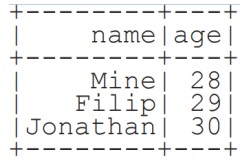From Spark Data Sources

JSON

 df = spark.read.json( "customer.json")
df.show()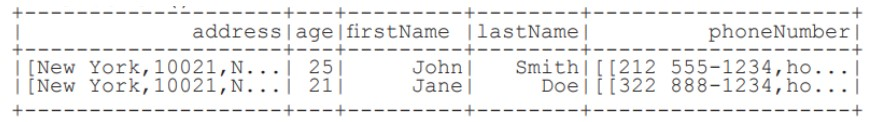df2 = spark.read.load( "people.json" , format= "json")


Parquet files

 df3 = spark.read.load("people.parquet" )


TXT files

 df4 = spark.read.text( "people.txt")


### Filter

Filter entries of age, only keep those records of which the values are >24

 df.filter(df["age"] >24).show()


Duplicate Values

 df = df.dropDuplicates()


### Queries

What is PySpark SQL? PySpark SQL is the most popular PySpark module that is used to process structured columnar data. Once a DataFrame is created, we can interact with data using the SQL syntax. Spark SQL is used for bringing native raw SQL queries on Spark by using select, where, group by, join, union etc. For using PySpark SQL, the first step is to create a temporary table on DataFrame by using createOrReplaceTempView()

 from pyspark.sql import functions as F


Select

 df.select( "firstName").show() #Show all entries in firstNome column
df.select( "firstName","lastName") \
.show()
df.select( "firstName", #Show all entries in firstNome, age and type
"age" ,
explode(''phoneNumber'') \
.alias(''contactlnfo')') \
.select("contactlnfo.type",
"firstName",
"age" ) \
.show()
df.select(df["firstName",df[ "age" ]+ 1)  #Show all entries in firstName and age,
.show()	#add 1 to the entries of age
df.select(df['age'] > 24).show() #Show all entries where age >24



When

 df.select( "firstName", #Show firstName and 0 or 1 depending on age >30
F.when(df.age > 30, 1) \
.otherwise(0)) \
.show()
df[ df.firstName.isin( "Jane" ,"Boris") ] #Show firstName if in the given options
.collect()


Like

#Show firstName, and lastName is TRUE if lastName is like Smith
df.select( "firstName",
df.lastName .like(''Smith')') \
.show()


Startswith - Endswith

 df.select( "firstName", #Show firstName, and TRUE if lastName starts with Sm
df.lastName \
.startswith("Sm")) \
.show()
df.select(df.lastName.endswith("th"))\ #Show last names ending in th
.show()



Substring

 df.select(df.firstName.substr(l, 3) \ #Return substrings of firstName
.alias(''name')') \
.collect()



Between

 df.select(df.age.between(22, 2s)) \ #Show age: values are TRUE if between 22 and 24


### Add, Update & Remove Columns

 df = df.withColumn( 'city',df.address.city) \
.withColumn( 'telePhoneNumber ',explode(df.phoneNumber.number)) \
.withColumn( 'telePhone Type',explode(df.phoneNumber.type))



Updating Columns

 df=df.withColumnRenamed('telePhoneNumber ','phoneNumber' )


Removing Columns

 df = df.drop ("address","phoneNumber")


### Missing & Replacing Values

 df.na.fill(50).show() #Replace null values
df.na.drop().shaw() #Return new df omitting rows with null values
df.na \ #Return new df replacing one value with another
.replace(10, 20) \
.show()


### GroupBy

 df.groupBy("age")\ #Group by age, count the members in the groups
.count() \
.show()


### Sort

 peopledf.sort(peopledf.age.desc()).collect()
df.sort("age" , ascending=False).collect()
df.orderBy([ "age", "city" ],ascending=[0,1])\
.collect()


### Repartitioning

 df.repartitian(10)\ #df with 10 partitions
.rdd \
.getNumPartitions()
df.coalesce(1).rdd.getNumPartitions() #df with 1 partition


### Running Queries Programmatically

 peopledf.createGlobalTempView( "people")
df.createTempView ("customer")
df.createOrReplaceTempView( "customer")


Query Views

 df5 = spark.sql("SELECT * FROM customer").show()
peopledf2=spark.sql( "SELEC T* FROM global_ temp.people")\
.show()


### Inspect Data

 df.dtypes #Return df column names and data types
df.show() #Display the content of df
df.first() #Return first row
df.take(2) #Return the first n rows
df.schema Return the schema of df
df.describe().show() #Compute summary statistics
df.columns Return the columns of df
df.count() #Count the number of rows in df
df.distinct().count() #Count the number of distinct rows in df
df.printSchema() #Print the schema of df
df.explain() #Print the (logical and physical) plans


### Output

Data Structures

 rddl = df.rdd #Convert df into an ROD
df.taJSON().first() #Convert df into a ROD of string
df.toPandas() #Return the contents of df as Pandas DataFrame


Write & Save to Files

 df.select( "firstName", "city")\
.write \
.save("nameAndCity.parquet" )
df.select("firstName", "age") \
.write \
.save( "namesAndAges.json",format="json")


### Stopping SparkSession

spark.stop()


## PySpark RDD

PySpark is the Spark Python API that exposes the Spark programming model to Python.

Inspect SparkContext

 sc.version #Retrieve SparkContext version
sc.pythonVer #Retrieve Python version
sc.master #Master URL to connect to
str(sc.sparkHome) #Path where Spark is installed an worker nodes
str(sc.sparkUser()) #Retrieve name of the Spark User running SparkContext
sc.appName #Return application name
sc.applicationld #Retrieve application ID
sc.defaultParallelism #Return default level of parallelism
sc.defaultMinPartitions #Default minimum number of partitions for RDDs



Configuration

 from pyspark import SparkConf, SparkContext
conf = (SparkConf()
.setMaster("local")
.setAppName("My app")
.set("spark.executor.memory","1g" ) )
sc = SparkContext(conf = conf)


Using The Shell

In the PySpark shell, a special interpreter aware SparkContext is already created in the variable called sc.

$./bin/spark shell --master local$ ./bin/pyspark --master local --py files code.py


Set which master the context connects to with the –master argument, and add Python .zip, .egg or .py files to the runtime path by passing a comma separated list to –py-files

Parallelized Collections

 rdd = sc.parallelize([('a',7),('a',2),('b',2)])
rdd = sc.parallelize([('a',2),('d',1),('b',1)])
rdd3 = sc.parallelize(range(100))
rdd4 = sc.parallelize([("a",["x","y","z"]),
("b",["p","r"])]


External Data

Read either one text file from HDFS.a local file system or or any Hadoop-supported file system URI with textFile(). or read in a directory of text files with wholeTextFiles()

 textFile = sc.textFile("/my/directory/*.txt")
textFile2 = sc.wholeTextFiles( "/my/directory/")


### Retrieving RDD Information

Basic Information

 rdd.getNumPartitions() #List the number of partitions
rdd.count() #Count ROD instances 3
rdd.countByKey() #Count ROD instances by key
defaultdict(<type 'int'>,{'a':2,'b' :1})
rdd.countByValue() #Count ROD instances by value
defaultdict(<type 'int'>,{('b',2):1,'(a',2):1,('a',7):1})
rdd.collectAsMap() #Return (key,value) pairs as a dictionary
{'a':2,1b':2}
rdd3.sum() #Sum of ROD elements 4950
sc.parallelize([]).isEmpty() #Check whether ROD is empty
True



Summary

 rdd3.max() #Maximum value of ROD elements 99
rdd3.min() #Minimum value of ROD elements
0
rdd3.mean() #Mean value of ROD elements
,9.5
rdd3.stdev() #Standard deviation of ROD elements 2a.8660700s772211a
rdd3.variance() #Compute variance of ROD elements 833.25
rdd3.histogram(3) #Compute histogram by bins
([0,33,66,991,[33,33,3,])
rdd3.stats() #Summary statistics (count, mean, stdev, max & min)



### Applying Functions

#Apply a function to each ROD element
rdd.map(lambda x: x+(x[l],x)).callect()
[('a',7,7,'a'),('a',2,2,'a'),('b',2,2,'b')]
#Apply a function to each ROD element and flatten the result
rdd5 = rdd.flatMap(lambda x: x+(x[l],x))
rdd5.collect()
['a',7,7'a','a',2,2'a','b',2,2'b']
#Apply a flatMap function to each (key,value) pair of rdd4 without changing the keys
rdds.flatMapValues(lambda x: x).callect()
[('a','x'),('a','y'),('a','z'),('b','p'),('b','r')]



### Selecting Data

Getting

 rdd.collect() #Return a list with all ROD elements
[('a',7),('a',2),('b',2)]
rdd.take(2) #Take first 2 ROD elements
[('a',7),('a',2)]
rdd.first() #Toke first ROD element
[('a',7),('a',2)]
rdd.top(2) #Take top 2 ROD elements
[('b',2),('a',7)]


Sampling

 rdd3.sample(False, 0.15, 81).collect() #Return sampled subset of rdd3
[3,4,27,31,40,41,42,43,60,76,79,80,86,97]


Filtering

 rdd.filter(lambda x: "a" in x).collect() #Filter the ROD
[( 'a',7),('a',2)]
rdd5.distinct().callect() #Return distinct ROD values
['a',2,'b',7]
rdd.keys().collect() #Return (key,value) RDD's keys
['a','a','b']


 def g(x): print(x)
rdd.foreach(g) #Apply a function to all ROD elements
('a',7)
('b',2)
('a',2)



### Reshaping Data

Reducing

 rdd.reduceByKey(lambda x,y : x+y).callect() #Merge the rdd values for each key
[('a',9),('b',2)]
rdd.reduce(lambda a, b: a+	b) #Merge the rdd values
('a',7,'a',2,'b',2)



Grouping by

 rdd3.groupBy(lambda x: x % 2)
.mapValues(list)
.collect()
rdd.groupByKey()
.mapValues(list)
.collect()
[('a',[7,2]),('b',)]


Aggregating

 seqOp = (lambda x,y: (x+y,x+1))
combOp = (lambda x,y:(x+y,x+y))
#Aggregate RDD elements of each partition and then the results
rdd3.aggregate((0,0),seqOp,combOp)
(4950,100)
#Aggregate values of each RDD key
rdd.aggregateByKey((0,0),seqop,combop).collect()
[('a',(9,2)),('b',(2,1))]
#Aggregate the elements of each partition, and then the results
4950
#Merge the values for each key
[('a',9),('b',2)]
#Create tuples of RDD elements by applying a function
rdd3.keyBy(lambda x: x+x).collect()


### Mathematical Operations

 rdd.subtract(rdd2).collect() #Return each rdd value not contained in rdd2
[('b',2),('a',7)]
#Return each (key,value) pair of rdd2 with no matching key in rdd
rdd2.subtractByKey(rdd).collect()
[('d',1)l
rdd.cartesian(rdd2).callect() #Return the Cartesian product of rdd and rdd2


Sort

 rdd2.sortBy(lambda x: x[l]).collect() #Sort ROD by given function
[('d',1),('b',1),('a',2)]
rdd2.sartByKey().collect() #Sort (key, value) ROD by key
[('a',2),('b',1),('d',1)]


Repartitioning

 rdd.repartitian(4) #New ROD with 4 partitions
rdd.caalesce(1) #Decrease the number of partitions in the ROD to 1



Saving

 rdd .saveA sTextFile("rdd.txt")



Execution

\$ ./bin/spark submit examples/src/main/python/pi.py


Does PySpark provide a machine learning API?

Similar to Spark, PySpark provides a machine learning API which is known as MLlib that supports various ML algorithms like:

• mllib.classification − This supports different methods for binary or multiclass classification and regression analysis like Random Forest, Decision Tree, Naive Bayes etc.
• mllib.clustering − This is used for solving clustering problems that aim in grouping entities subsets with one another depending on similarity.
• mllib.fpm − FPM stands for Frequent Pattern Matching. This library is used to mine frequent items, subsequences or other structures that are used for analyzing large datasets.
• mllib.linalg − This is used for solving problems on linear algebra.
• mllib.recommendation − This is used for collaborative filtering and in recommender systems.
• spark.mllib − This is used for supporting model-based collaborative filtering where small latent factors are identified using the Alternating Least Squares (ALS) algorithm which is used for predicting missing entries.
• mllib.regression − This is used for solving problems using regression algorithms that find relationships and variable dependencies.
• Is PySpark faster than pandas?

PySpark supports parallel execution of statements in a distributed environment, i.e on different cores and different machines which are not present in Pandas. This is why PySpark is faster than pandas.

Broadcast variables: These are also known as read-only shared variables and are used in cases of data lookup requirements. These variables are cached and are made available on all the cluster nodes so that the tasks can make use of them. The variables are not sent with every task. They are rather distributed to the nodes using efficient algorithms for reducing the cost of communication. When we run an RDD job operation that makes use of Broadcast variables, the following things are done by PySpark:

The job is broken into different stages having distributed shuffling. The actions are executed in those stages. The stages are then broken into tasks. The broadcast variables are broadcasted to the tasks if the tasks need to use it. Broadcast variables are created in PySpark by making use of the broadcast(variable) method from the SparkContext class. The syntax for this goes as follows:

broadcastVar = sc.broadcast([10, 11, 22, 31])


An important point of using broadcast variables is that the variables are not sent to the tasks when the broadcast function is called. They will be sent when the variables are first required by the executors.

What is Accumulator variable?

Accumulator variables: These variables are called updatable shared variables. They are added through associative and commutative operations and are used for performing counter or sum operations. PySpark supports the creation of numeric type accumulators by default. It also has the ability to add custom accumulator types. The custom types can be of two types:

What is PySpark Architecture?

PySpark similar to Apache Spark works in master-slave architecture pattern. Here, the master node is called the Driver and the slave nodes are called the workers. When a Spark application is run, the Spark Driver creates SparkContext which acts as an entry point to the spark application. All the operations are executed on the worker nodes. The resources required for executing the operations on the worker nodes are managed by the Cluster Managers

What is the common workflow of a spark program?

The most common workflow followed by the spark program is: The first step is to create input RDDs depending on the external data. Data can be obtained from different data sources. Post RDD creation, the RDD transformation operations like filter() or map() are run for creating new RDDs depending on the business logic. If any intermediate RDDs are required to be reused for later purposes, we can persist those RDDs. Lastly, if any action operations like first(), count() etc are present then spark launches it to initiate parallel computation.

Congratulations! You have read an small summary about important things in Data Science.

Posted: